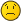Real and complex tetrationDanielLong Time FellowPosts: 279 Threads: 94 Joined: Aug 2007 06/21/2022, 03:24 AM (This post was last modified: 06/21/2022, 04:26 AM by Daniel.) My approach to fractional tetration involves the complex numbers. Others have found fractional tetration constrained to the real numbers to be more natural. Which tetration methods require complex numbers and which only need real numbers? For example I consider Kneser as real based because fractional tetration of f(x) where $f(f(x))=e^x$ is real even though the proof uses complex numbers. DanielJmsNxnUltimate FellowPosts: 1,214 Threads: 126 Joined: Dec 2010 06/21/2022, 10:19 PM (06/21/2022, 03:24 AM)Daniel Wrote: My approach to fractional tetration involves the complex numbers. Others have found fractional tetration constrained to the real numbers to be more natural. Which tetration methods require complex numbers and which only need real numbers? For example I consider Kneser as real based because fractional tetration of f(x) where $f(f(x))=e^x$ is real even though the proof uses complex numbers. I don't know of any standard of terms for this. But complex valued tetration would be what your tetration would be called. And real valued tetration would be a tetration that preserves real values. A real valued tetration can still produce complex numbers, but only for complex arguments. A complex valued tetration can produce complex numbers for real arguments. With the exception that we ignore $(-\infty,-2]$, which produces complex values for Kneser; but that's okay because it's not analytic here. I think a standard way to phrase this would be real-valued iteration, versus a complex valued iteration. Your question is very hard to answer because there are uncountably infinitely many tetrations, lol. So to ask which are real valued and which are not is actually a very deep problem. Especially if you no longer ask for analycity, and solely talk about smooth solutions (on the real line). It's widely conjectured the only real-valued tetration with $b > \eta$ and holomorphic in the upper half plane is Kneser. This procedure fails within Shell-Thron $e^{-e} \le b \le \eta$ and there are uncountably infinite real-valued tetrations--with uniqueness criterion depending on what qualities you want of your iteration. Generally, the hardest part about tetration is A.) making it real valued, B.) seeing if it's the same real-valued solution as another/Uniqueness. Not sure what else can be saidGottfriedUltimate FellowPosts: 901 Threads: 130 Joined: Aug 2007 06/22/2022, 10:52 AM (06/21/2022, 10:19 PM)JmsNxn Wrote: (...)  But complex valued tetration would be what your tetration would be called. And real valued tetration would be a tetration that preserves real values. A real valued tetration can still produce complex numbers, but only for complex arguments. A complex valued tetration can produce complex numbers for real arguments. (...) Hi buddie ( ;-) ) -  well said.    My most liked example is that of the fractional interpolation of the fibonacci-numbers.     - Do we want to be real to real?  - Do we want to be real to complex? And then: why? The -for me- most natural interpolation is that along the Binet-formula. But this gives real-to-complex interpolation.  In wikipedia one finds the real-to-real interpretation, which is in my view an ugly hoax, siimply introducing the $\cos()$-function to distort the real-to-complex way.  (a small discussion as a result of a random observation in a discussion of fractional iteration of $f(x)={1 \over 1+x}$, see plot-example in section 5) Over the long run, I think, the decision shall be made once we observe a fibonacci-process in nature and have as well reason to assume that the interpolated process is in nature as well. Before that might occur, I think we can only decide in terms of (mathematical) elegance, versatility, ... . Well, this is a toy model, but it might sharpen the mind for the argumentation when we insert our problem "tetration" instead the term "fibonacci" and ask for real-to-complex or real-to-real-interpolation method ... Gottfried Gottfried Helms, Kasseltommy1729Ultimate FellowPosts: 1,924 Threads: 415 Joined: Feb 2009 06/22/2022, 11:16 PM I also mentioned this fibonacci type idea earlier. see :  https://math.eretrandre.org/tetrationfor...=fibonacci In particular reducing the recursion to an iteration to fit dynamics better : ratfibo(x+1) = 1 + 1/ratfibo(x)  with ratfibo the ratio of consecutive fibo numbers. Thus reducing to iterations where there are 2 fixpoints. Also mike 3 made a nice contribution here  https://math.eretrandre.org/tetrationfor...=fibonacci I think there are still things to talk about. is going from iterations to recursions the next step ?? Is there a kneser like fibonacci ? Many questions regards tommy1729 « Next Oldest | Next Newest »

 Possibly Related Threads… Thread Author Replies Views Last Post Behaviour of tetration into the real negatives Shanghai46 1 349 10/12/2023, 11:01 PM Last Post: leon Real tetration as a limit of complex tetration Daniel 6 947 10/10/2023, 03:23 PM Last Post: leon Real and complex tetration Daniel 13 3,025 04/04/2023, 10:25 AM Last Post: JmsNxn Evaluating Arithmetic Functions In The Complex Plane Caleb 6 1,282 02/20/2023, 12:16 AM Last Post: tommy1729 Range of complex tetration as real Daniel 2 1,017 10/22/2022, 08:08 PM Last Post: Shanghai46 From complex to real tetration Daniel 3 1,193 10/21/2022, 07:55 PM Last Post: Daniel Cost of real tetration Daniel 1 851 09/30/2022, 04:41 PM Last Post: bo198214 Real Multivalued/Parametrized Iteration Groups bo198214 11 3,031 09/10/2022, 11:56 AM Last Post: tommy1729 Constructive real tetration Daniel 1 773 09/01/2022, 05:41 AM Last Post: JmsNxn What are the types of complex iteration and tetration? Daniel 5 1,738 08/17/2022, 02:40 AM Last Post: JmsNxn

Users browsing this thread: 1 Guest(s)